# Test: Sequences And Series - 1

## 15 Questions MCQ Test Practice Questions for GMAT | Test: Sequences And Series - 1

Description
Attempt Test: Sequences And Series - 1 | 15 questions in 30 minutes | Mock test for GMAT preparation | Free important questions MCQ to study Practice Questions for GMAT for GMAT Exam | Download free PDF with solutions
QUESTION: 1

### If integer k is equal to the sum of all even multiples of 15 between 295 and 615, what is the greatest prime factor of k?

Solution:

First, let us simplify the problem by rephrasing the question. Since any even number must be divisible by 2, any even multiple of 15 must be divisible by 2 and by 15, or in other words, must be divisible by 30. As a result, finding the sum of even multiples of 15 is equivalent to finding the sum of multiples of 30. By observation, the first multiple of 30 greater than 295 will be equal to 300 and the last multiple of 30 smaller than 615 will be equal to 600.

Thus, since there are no multiples of 30 between 295 and 299 and between 601 and 615, finding the sum of all multiples of 30 between 295 and 615, inclusive, is equivalent to finding the sum of all multiples of 30 between 300 and 600, inclusive. Therefore, we can rephrase the question: “What is the greatest prime factor of the sum of all multiples of 30 between 300 and 600, inclusive?”

The sum of a set = (the mean of the set) × (the number of terms in the set)

Since 300 is the 10th multiple of 30, and 600 is the 20th multiple of 30, we need to count all multiples of 30 between the 10th and the 20th multiples of 30, inclusive.
There are 11 terms in the set: 20th – 10th + 1 = 10 + 1 = 11
The mean of the set = (the first term + the last term) divided by 2: (300 + 600) / 2 = 450
k = the sum of this set = 450 × 11

Note, that since we need to find the greatest prime factor of k, we do not need to compute the actual value of k, but can simply break the product of 450 and 11 into its prime factors:
k = 450 × 11 = 2 × 3 × 3 × 5 × 5 × 11

Therefore, the largest prime factor of k is 11.

QUESTION: 2

### If S is the infinite sequence S1 = 6, S2 = 12, ..., Sn = Sn-1 + 6,..., what is the sum of all terms in the set {S13, S14, ..., S28}?

Solution:

For sequence S, any value Sn equals 6n. Therefore, the problem can be restated as determining the sum of all multiples of 6 between 78 (S13) and 168 (S28), inclusive. The direct but time-consuming approach would be to manually add the terms: 78 + 84 = 162; 162 + 90 = 252; and so forth.

The solution can be found more efficiently by identifying the median of the set and multiplying by the number of terms.  Because this set includes an even number of terms, the median equals the average of the two ‘middle’ terms, S20 and S21, or (120 + 126)/2 = 123. Given that there are 16 terms in the set, the answer is 16(123) = 1,968.

QUESTION: 3

### In an increasing sequence of 5 consecutive even integers, the sum of the second, third, and fourth integer is 132. What is the sum of the first and last integers?

Solution:

Let the five consecutive even integers be represented by x, x + 2, x + 4, x + 6, and x + 8. Thus, the second, third, and fourth integers are x + 2, x + 4, and x + 6. Since the sum of these three integers is 132, it follows that

3x + 12 = 132, so
3x = 120, and
x = 40.

The first integer in the sequence is 40 and the last integer in the sequence is x + 8, or 48.

The sum of 40 and 48 is 88.

QUESTION: 4

What is the sum of the multiples of 7 from 84 to 140, inclusive?

Solution:

84 is the 12th multiple of 7. (12 x 7 = 84)
140 is the 20th multiple of 7.
The question is asking us to sum the 12th through the 20th multiples of 7.
The sum of a set = (the mean of the set) x (the number of terms in the set)
There are 9 terms in the set: 20th - 12th + 1 = 8 + 1 = 9
The mean of the set = (the first term + the last term) divided by 2:  (84 + 140)/2 = 112
The sum of this set = 112 x 9 = 1008
Alternatively, one could list all nine terms in this set (84, 91, 98 ... 140) and add them.
When adding a number of terms, try to combine terms in a way that makes the addition easier
(i.e. 98 + 112 = 210, 119 + 91 = 210, etc).

QUESTION: 5

If each term in the sum a1 + a2 + a3 + ... +an is either 7 or 77 and the sum is equal to 350, which of the
following could equal to n?

Solution:
QUESTION: 6

For any integer k from 1 to 10, inclusive, the kth of a certain sequence is given by [(-1)(k+1)] × (1 / 2k). If T is the sum of the first 10 terms of the sequence, then T is:

Solution:

T= 1/2-1/22+1/23-...-1/210
= 1/4+1/42+1/43+1/44+1/45
Notice that 1/42+1/43+1/44+1/45 < 1/4, we can say that 1/4<T<1/2.

QUESTION: 7

S is the infinite sequence S1 = 2, S2 = 22, S3 = 222,...Sk = Sk–1 + 2(10k–1). If p is the sum of the first 30 terms of S, what is the eleventh digit of p, counting right to left from the units digit?

Solution:

The first few terms of the sequence are 2, 22, and 222 and each subsequent term has an additional 2 added on. The 30th term then is a string of 30 2’s. If we line up the first 30 terms of the sequence to add them up, we will get rows in the following pattern: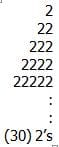To find p, the sum of the first 30 terms of S, we would simply be adding columns of 2’s. The key here is to see a pattern in the addition process. Starting with the units digit column, all 30 of the terms have a 2 in that position so the sum of the units column would be 30 x 2 = 60. A zero would be written as the units digit of the sum and a six would be carried over to the tens column.

In the tens column, 29 of the 30 terms would have a 2 because the first term has no tens digit. The sum of the tens digits would be 29 x 2 = 58, to which we must add the 6 for a total of 64. The 4 gets written down as the second digit of p and the 6 is carried over to the hundreds column.

In the hundreds column, 28 of the 30 terms would have a 2, the sum of the hundreds digits would be 28 x 2 = 56, to which we must add the 6 again for a total of 62.   The 2 gets written down as the third digit of p and the 6 is carried over to the thousands column.

There are two ways to finish this problem. We can do out the remaining 8 columns and find that the 11th digit (i.e. the 10 billions column) will have a sum of 2(20) + 4 = 44 (where the 4 was carried over from the 10th column).  4 then will be the 11th digit (from the right) of p (and a 4 will be carried over into the 12th column).

We could also have seen that each column has one less 2 than the previous, so if we started out with 30 2’s in the first column, the 11th column must have 11 – 1 = 10 less 2’s, for a total of 20 2’s. The amount that is carried over from the previous column could be calculated by realizing that the 10th column had 21 2’s for a total of 42. Since there is no way that the 10th column inherited more than 8 from the 9th column, the total must be forty-something and the amount that is carried over to the 11th column MUST BE 4. This makes the total for the 11th column 40 + 4 = 44 and the 11th digit of p4.

QUESTION: 8

Sequence S is defined as Sn = 2Sn-1 – 2. If S1 = 3, then S10 – S9 =

Solution:

We can use the formula to calculate the first 10 values of S:

S1 = 3
S2 = 2(3) – 2 = 4
S3 = 2(4) – 2 = 6
S4 = 2(6) – 2 = 10
S5 = 2(10) – 2 = 18
S6 = 2(18) – 2 = 34
S7 = 2(34) – 2 = 66
S8 = 2(66) – 2 = 130
S9 = 2(130) – 2 = 258
S10 = 2(258) – 2 = 514

S10 – S9 = 514 – 258 = 256.

Alternatively, we could solve this problem by noticing the following pattern in the sequence:
S2 – S1 = 1 or (20)
S3 – S2 = 2  or (21)
S4 – S3 = 4  or (22)
S5 – S4 = 8  or (23)

We could extrapolate this pattern to see that S10  S9 = 28 = 256.

QUESTION: 9

Sn = 2Sn-1 + 4 and Qn = 4Qn-1 + 8 for all n > 1. If S5 = Q4 and S7 = 316, what is the first value of n for whichQn is an integer?

Solution:

If S7 = 316, then 316 = 2S6 + 4, which means that S6=156.

We can then solve for S5
156 = 2S5 + 4, so S5 = 76

Since S5 = Q4, we know that Q4 = 76 and we can now solve for previous Qn’s to find the first n value for which Qn is an integer.

To find Q3: 76 = 4Q3 + 8, so Q3 = 17
To find Q2:  17 = 4Q2 + 8, so Q2 = 9/2
It is clear that Q1 will also not be an integer so there is no need to continue.

Q3 (n = 3) is the first integer value.

QUESTION: 10

What is the sixtieth term in the following sequence? 1, 2, 4, 7, 11, 16, 22...

Solution:

Noting that a1 = 1, each subsequent term can be calculated as follows:
a1 = 1
a2 = a1 + 1
a3 = a1 + 1 + 2
a4 = a1 + 1 + 2 + 3
ai = a1 + 1 + 2 + 3 + ... + i-1

In other words, ai = a1 plus the sum of the first i - 1 positive integers. In order to determine the sum of the first i - 1 positive integers, find the sum of the first and last terms, which would be 1 and i - 1 respectively, plus the sum of the second and penultimate terms, and so on, while working towards the median of the set. Note that the sum of each pair is always equal to i:

1 + (i - 1) = i
2 + (i – 2) = i
3 + (i – 3) = i
…

Because there are (i - 1)/2 such pairs in a set of i - 1 consecutive integers, this operation can be summarized by the formula i(i - 1)/2. For this problem, substituting a1 = 1 and using this formula for the sum of the first (i-1) integers yields:
ai = 1 + (i)(i - 1)/2

The sixtieth term can be calculated as:
a60 = 1 + (59)(60)/2
a60 = 1,771

QUESTION: 11

Sequence S is defined as Sn = X + (1/X), where X = Sn – 1 + 1, for all n > 1.
If S1= 201, then which of the following must be true of Q, the sum of the first 50 terms of S?

Solution:

To find each successive term in S, we add 1 to the previous term and add this to the reciprocal of the previous term plus 1.
S1= 201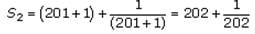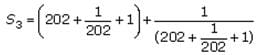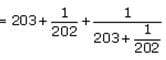The question asks to estimate (Q), the sum of the first 50 terms of S. If we look at the endpoints of the intervals in the answer choices, we see have quite a bit of leeway as far as our estimation is concerned. In fact, we can simply ignore the fractional portion of each term. Let’s use S2 ≈ 202, S3 ≈ 203. In this way, the sum of the first 50 terms of S will be approximately equal to the sum of the fifty consecutive integers 201, 202 … 250.
To find the sum of the 50 consecutive integers, we can multiply the mean of the integers by the number of integers since average = sum / (number of terms).
The mean of these 50 integers = (201 + 250) / 2 = 225.5
Therefore, the sum of these 50 integers = 50 x 225.5 = 11,275, which falls between 11,000 and 12,000. The correct answer is C.

QUESTION: 12

In a certain sequence, every term after the first is determined by multiplying the previous term by an integerconstant greater than 1. If the fifth term of the sequence is less than 1000, what is the maximum number ofnonnegative integer values possible for the first term?

Solution:

The equation of the sequence can be written as follows: an= (an-1 )(x) , where x is the integer constant.  So for every term after the first, multiply the previous term by x.  Essentially, then, all we are doing is multiplying the first term by x over and over again.  For example, (a2)= (a1)(x) and (a3)=(a2)(x) or a3 = ((a1)(x))(x), which is the same as (a3) = (a1)(x2)
If we keep going, we’ll see that  a3 = (a1)(x2) and so on for the rest of the sequence.  We can thus rewrite the equation of the sequence as an = (a1)(xn-1) , for all n >1.
We also know from the question that  a5 < 1000, which means that (a1)(x4) < 1000
We are asked for the maximum number of possible nonnegative integer values for  ; we can get this by minimizing the value of the integer constant, x. Since x is an integer constant greater than 1, the smallest possible value for x is 2. When x = 2, then x4= 16
We can solve for  a1 as follows: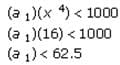Thus all the integers from 1 to 62, inclusive, are permissible for a1. So far we have 62 permissible values. If a1=0 then it doesn’t matter what x is, since every term in the sequence will always be 0.  So 0 is one more permissible value for a1
There is a maximum of 62 +1 (or 63) nonnegative integer values for ain which a5 < 1000.

QUESTION: 13

The sum of the squares of the first 15 positive integers (12 + 22 + 32 + . . . + 152) is equal to 1240. What is the sum of the squares of the second 15 positive integers (162 + 172 + 182 + . . . + 302) ?

Solution:

The key to solving this problem is to represent the sum of the squares of the second 15 integers as follows: (15 + 1)2 + (15 + 2)2 + (15 + 3)2 + . . . + (15 + 15)2.

Recall the popular quadratic form, (a + b)2 = a2 + 2ab + b2. Construct a table that uses this expansion to calculate each component of each term in the series as follows: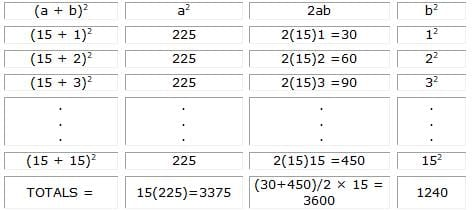In order to calculate the desired sum, we can find the sum of each of the last 3 columns and then add these three subtotals together. Note that since each column follows a simple pattern, we do not have to fill in the whole table, but instead only need to calculate a few terms in order to determine the sums.

The column labeled a2 simply repeats 225 fifteen times; therefore, its sum is 15(225) = 3375.

The column labeled 2ab is an equally spaced series of positive numbers. Recall that the average of such a series is equal to the average of its highest and lowest values; thus, the average term in this series is (30 + 450) / 2 = 240. Since the sum of n numbers in an equally spaced series is simply n times the average of the series, the sum of this series is 15(240) = 3600.

The last column labeled b2 is the sum of the squares of the first 15 integers. This was given to us in the problem as 1240.

Finally, we sum the 3 column totals together to find the sum of the squares of the second 15 integers: 3375 + 3600 + 1240 = 8215. The correct answer choice is (D).

QUESTION: 14

The infinite sequence Sk is defined as Sk = 10 Sk – 1 + k, for all k > 1. The infinite sequence An is defined as An = 10 An – 1 + (A1 – (n - 1)), for all n > 1. q is the sum of Sk and An. If S1 = 1 and A1 = 9, and if An is positive, what is the maximum value of k + n when the sum of the digits of q is equal to 9?

Solution:

In complex sequence questions, the best strategy usually is to look for a pattern in the sequence of terms that will allow you to avoid having to compute every term in the sequence.

In this case, we know that the first term of Sk is 1 and the first term of An  is 9. So when n = 1 and k = 1, q = 9 + 1 = 10 and the sum of the digits of q is 1 + 0 = 1.

since S1= 1, S2= (10)(1) +(2) = 10+2 = 12. since A1 =9,
A2= (10) (9)+(9-(2-1)) = 90+(9-1) = 90+8 = 98. so When K=2 and n = 2, q = 12 + 98 = 110 and the sum of the digits of q is 1 + 1 + 0 = 2.
Since S2= 12, S3 =(10)(12)+3= 120+3= 123. Since A2= 98, It is true that
A3= (10)(98) + (9-(3-1)) = 980+7 = 987. So when n = 3 and k = 3, q = 123 + 987 = 1110 and the sum of the digits of q is 1 + 1 + 1 + 0 = 3.
At this point, we can see a pattern: sproceeds as 1, 12, 123, 1234..., and An proceeds as 9, 98, 987, 9876.... The sum q therefore proceeds as 10, 110, 1110, 11110...  The sum of the digits of q, therefore, will equal 9 when q consists of nine ones and one zero. Since the number of ones in q is equal to the value of n and k (when n and k are equal to each other), the sum of the digits of q will equal 9 when n = 9 and k = 9:
S9 = 123456789 and A9 = 987654321. By way of illustration: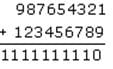When n > 9 and k > 9, the sum of the digits of q is not equal to 9 because the pattern of 10, 110, 1110..., does not hold past this point and the additional digits in q will cause the sum of the digits of q to exceed 9.
Therefore, the maximum value of k + n (such that the sum of the digits of q is equal to 9) is 9 + 9 = 18.

QUESTION: 15

A certain club has exactly 5 new members at the end of its first week. Every subsequent week, each
of the previous week's new members (and only these members) brings exactly x new members into the club. If y is the number of new members brought into the club during the twelfth week, which of the following could be y?

Solution:

At the end of the first week, there are 5 members.  During the second week, 5x new members are brought in (x new members for every existing member).  During the third week, the previous week's new members (5x) each bring in x new members:
(5x)x= 5xnew members.  If we continue this pattern to the twelfth week, we will see that 5x11 new members join the club that week.  Since y is the number of new members joining during week 12, y=5x11.
if y=5x11, we can set each of the answer choices equal to 5x11 and see which one yields an integer value (since y is a specific number of people, it must be an integer value).  The only choice to yield an integer value is (D)
5x11= 311512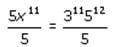x11 = 311512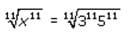x=(3)(5)
Therefore x = 15.
Since choice (D) is the only one to yield an integer value, it is the correct answer.Use Code STAYHOME200 and get INR 200 additional OFF Use Coupon Code